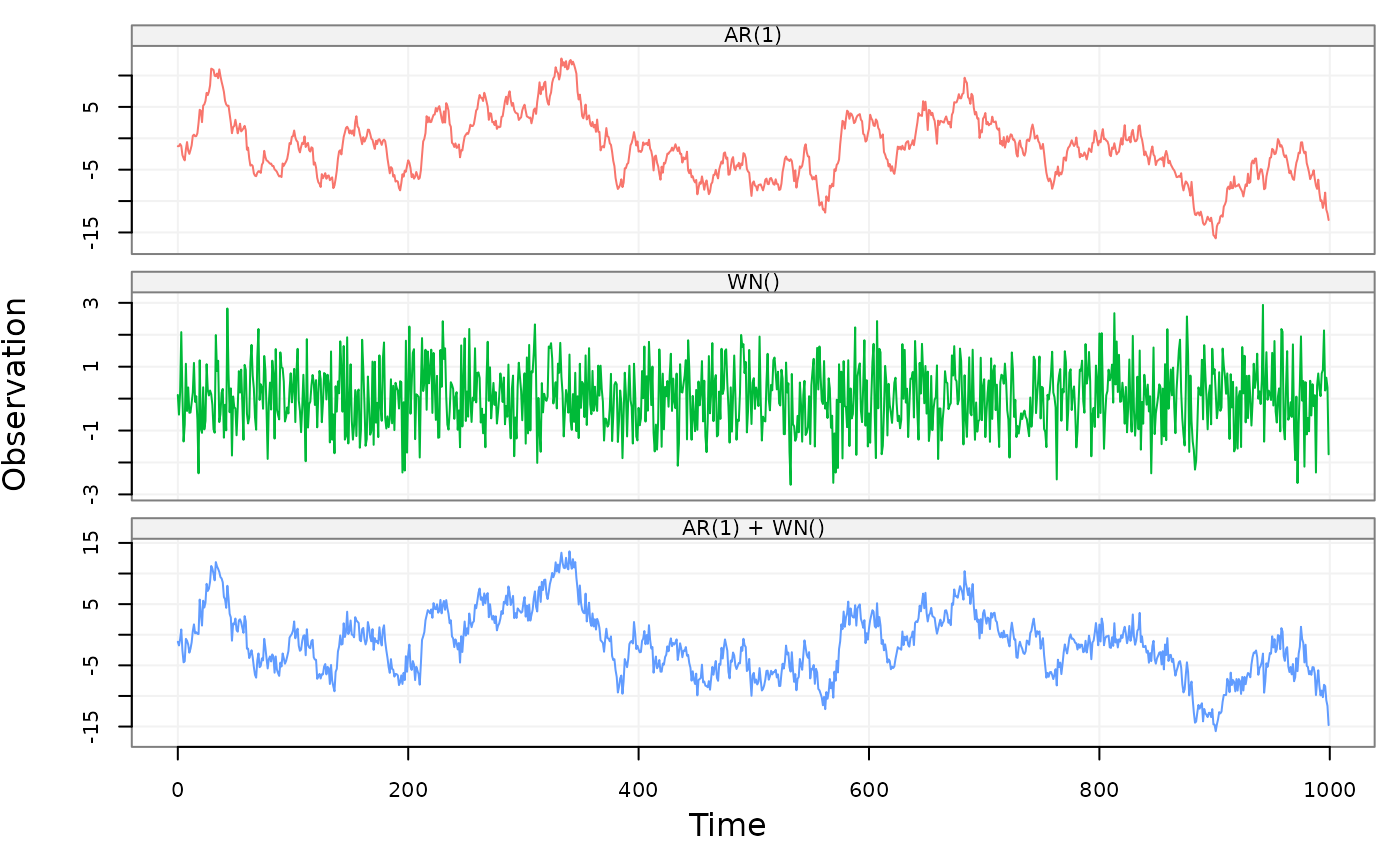Simulate a lts object based on a supplied time series model.

gen_lts(
n,
model,
start = 0,
end = NULL,
freq = 1,
unit_ts = NULL,
unit_time = NULL,
name_ts = NULL,
name_time = NULL,
process = NULL
)

## Arguments

n

An interger indicating the amount of observations generated in this function.

model

A ts.model or simts object containing one of the allowed models.

start

A numeric that provides the time of the first observation.

end

A numeric that provides the time of the last observation.

freq

A numeric that provides the rate/frequency at which the time series is sampled. The default value is 1.

unit_ts

A string that contains the unit of measure of the time series. The default value is NULL.

unit_time

A string that contains the unit of measure of the time. The default value is NULL.

name_ts

A string that provides an identifier for the time series data. Default value is NULL.

name_time

A string that provides an identifier for the time. Default value is NULL.

process

A vector that contains model names of each column in the data object where the last name is the sum of the previous names.

## Value

A lts object with the following attributes:

start

The time of the first observation.

end

The time of the last observation.

freq

Numeric representation of the sampling frequency/rate.

unit

A string reporting the unit of measurement.

name

Name of the generated dataset.

process

A vector that contains model names of decomposed and combined processes

## Details

This function accepts either a ts.model object (e.g. AR1(phi = .3, sigma2 =1) + WN(sigma2 = 1)) or a simts object.

## Author

James Balamuta, Wenchao Yang, and Justin Lee

## Examples

# AR
set.seed(1336)
model = AR1(phi = .99, sigma2 = 1) + WN(sigma2 = 1)
test = gen_lts(1000, model)
plot(test)